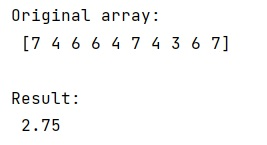# How do you find the IQR in NumPy?

Learn, how to find the IQR in NumPy in Python?
Submitted by Pranit Sharma, on January 12, 2023

NumPy is an abbreviated form of Numerical Python. It is used for different types of scientific operations in python. Numpy is a vast library in python which is used for almost every kind of scientific or mathematical operation. It is itself an array which is a collection of various methods and functions for processing the arrays.

## Finding the IQR in NumPy

IQR is Interquartile Range and Quartile Deviation. A quartile cam be considered as a quantile. The first quartile (Q1), is the number present between the smallest number and the median of the data set, the second quartile (Q2) is the median of the given data set, and the third quartile (Q3), is the middle value between the median and the largest value of the data set.

The range can be defined as the difference between the largest value and the smallest value in the given data set.

For this purpose, we will calculate multiple percentiles, and then we will find the interpercentile difference.

Let us understand with the help of an example,

## Python code to find the IQR in NumPy

```# Import numpy
import numpy as np

# Creating a numpy array
arr = np.array([7,4,6,6,4,7,4,3,6,7])

# Display original array
print("Original array:\n",arr,"\n")

# Calculating percentile
p75, p25 = np.percentile(arr, [75 ,25])

# Finding iqr
res = p75 - p25

# Display result
print("Result:\n",res,"\n")
```

Output: